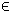## 19MINIMAXLIN Problem

The linear minimax (mimalin) problem is defined as
 min x
maxDx
subject to xL x xU
bL Ax bU
(21)
where x,xL,xURn, bL,bURm1, ARm1 × n and DRm2 × n.

The following file illustrates how to solve a linear minimax problem in TOMLAB.

File: tomlab/quickguide/minimaxlinQG.m

Open the file for viewing, and execute minimaxlinQG in Matlab.
```% minimaxlinQG is a small example problem for defining and solving
% linear minimax programming problems using the TOMLAB format.

Name = 'Linear Minimax Test 1';
x_0  = [1;1;1;1];          % Initial value
x_L  = [-10;-10;-10;-10];  % Lower bounds on x
x_U  = [10;10;10;10];      % Upper bounds on x

% Solve the problem min max Dx while eliminating abs for the final two
% residuals by adding them with reverse signs.
% i.e. min max [D_1; D_2; D_3; -D_2; -D_3];
D = [9 8 7 6; -4 5 -6 -2; 3 4 5 -6; 4 -5 6 2; -3 -4 -5 6]; % D Matrix

% Add the linear constraint -x(1) + x(2) + 2 >= 0
% Write the constraint as x(1) - x(2) <= 2

% The A matrix could be specified dense or sparse
% A   = sparse([1 -1 0 0]);

A   = [1 -1 0 0];
b_L = -inf;
b_U = 2;

c = zeros(4,1); % Dummy objective

% Generate an LP problem using the Tomlab Quick format
% Use mipAssign if solving a mixed-integer problem
Prob = lpAssign(c, A, b_L, b_U, x_L, x_U, x_0, Name);
Prob.QP.D = D;

Prob.f_Low = 0;
Prob.SolverInf = 'minos';

% One may set other solvers:
% Prob.SolverInf = 'cplex';
% Prob.SolverInf = 'xa';
% Prob.SolverInf = 'snopt';
% Prob.SolverInf = 'milpSolve';

% Set print level 1 to get output from PrintResult at the end
PriLev = 1;
Prob.PriLevOpt = 0;

Result  = tomRun('infLinSolve', Prob, PriLev);```